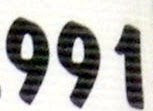## Monday, March 1, 2010

### 991

991 is a prime number.

991 is the largest three-digit permutable prime. In other words, 991, 919, and 199 are all primes.

991 is a number that cannot be written as a sum of three squares.

991 is the maximum number of regions into which 44 lines divide a plane.

9912 = 982081 and 982 + 0 + 8 + 1 = 991.Florida angler Allen Ogle caught a 991-pound hammerhead shark May 30, 1982.